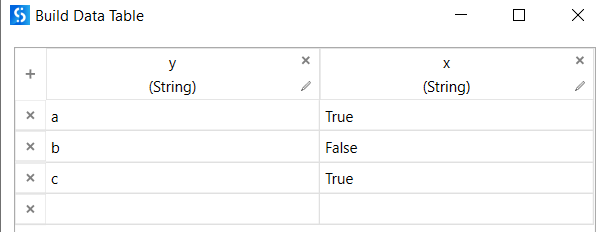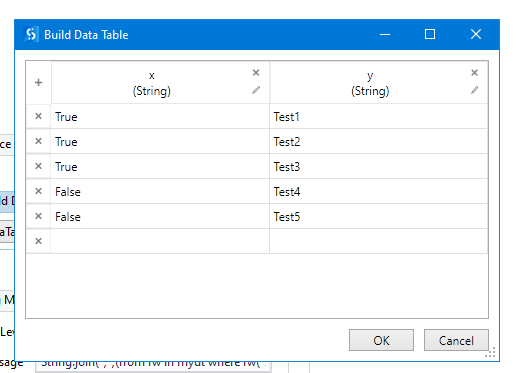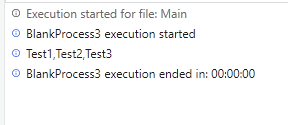# Data tables

this is actually a question on linq but im working with ui path so i thought its suits here,
i have a data table with two columns x of bolean and y of strings
i want to concat to a string separated by a , of all the values on column y where the cloumn x is true im trying to do this with Linq and with mydt.where(function(r) r(“x”).equals(“True”)) and now i dont know how to continue with the select funcion can someone help me please?
thanks

i cant edit the topic i dont know why but i meant to write mydt.asenumerable().where(function(r) r(“x”).equals(“True”))

this should work fine for you
`String.join(",",(from rw in mydt where rw("x").equals("True") select x = rw("y").ToString()).ToList)`

Here is a video with LINQ to get data from DataTables. Maybe it could help you further: https://www.youtube.com/watch?v=Z6AewjSuC1Y

Best regards
Mahmoud

i get an error while trying to run this command, why did you add the “x=”?
the error is just “cannot assign this paramter” and im trying to assign it to a string parameter

Example`String.Join(","c, mydt.AsEnumerable.Where(function(row) row.Field(Of String)("x").Equals("True")).Select(function(row) row.Field(Of String)("y")).ToArray)`

Resultworking perfectly fine for me.if possible can you upload your xaml. It could be variable data type issue.

x in query is range variable more info Working with Range Variables and Let Statements in LINQ | Developer.com